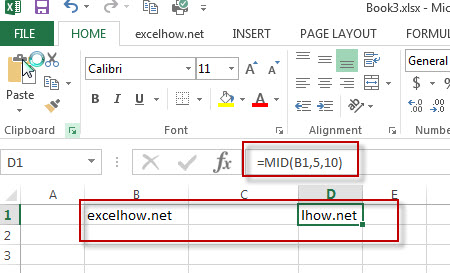# Excel Mid Function

This post will guide you how to use Excel MID function with syntax and examples in Microsoft excel.

## Description

The Excel MID function returns a substring from a text string at the position that you specify.

The MID function is a build-in function in Microsoft Excel and it is categorized as a Text Function.

The MID function is available in Excel 2016, Excel 2013, Excel 2010, Excel 2007, Excel 2003, Excel XP, Excel 2000, Excel 2011 for Mac.

## Syntax

The syntax of the MID function is as below:

`= MID (text, start_num, num_chars)`

Where the MID function arguments are:

• text -This is a required argument. The text string that you want to extract substring from.
• start_num-This is a required argument.  The position of the first character that you want to extract in text string.
• num_chars-This is a required argument.  The number of the characters that you want to extract from a text string.

Note:

• If start_num is greater than the length of text, the MID function will return empty text.
• If start_num is less than 1, the MID function will return the #VALUE! Error value.
• If num_chars is negative, MID will return the #VALUE!  Error value.

## Excel MID Function Example

The below examples will show you how to use Excel MID Text function to extract a substring from a text string.

#1 To extract 10 characters from the text string in B1, starting at the 5th character, just using the following formula:

`=MID(B1,5,10)`### More Excel MID Formula Examples

• Remove Numeric Characters from a Cell
If you want to remove numeric characters from alphanumeric string, you can use the following complex array formula using a combination of the TEXTJOIN function, the MID function, the Row function, and the INDIRECT function..…
• Split Text String to an Array
If you want to convert a text string into an array that split each character in text as an element, you can use an excel formula to achieve this result. the below will guide you how to use a combination of the MID function, the ROW function, the INDIRECT function and the LEN function to split a string…
• Remove non numeric characters from a cell
If you want to remove non numeric characters from a text cell in excel, you can use the array formula:{=TEXTJOIN(“”,TRUE,IFERROR(MID(B1,ROW(INDIRECT(“1:”&LEN(B1))),1)+0,””))}
• Get the position of Last Occurrence
If you want to get the position of the last occurrence of a character in a cell, then you can use a combination of the LOOKUP function, the MID function, the ROW function, the INDIRECT function and the LEN function to create an excel formula…
• Convet Text Date dd/mm/yy to mm/dd/yyyy as Date Format
If you want to convert dates in the text format to the date format as mm/dd/yyyy, you can use a combination of the DATE function, the VALUE function, the RIGHT function, the MID function, and the LEFT function to create a new excel formula…
• Split Text and Numbers
If you want to split text and numbers, you can run the following excel formula that use the MIN function, FIND function and the LEN function within the LEFT function in excel. And if you want to extract only numbers within string, then you also need to RIGHT function to combine with the above functions..…
• Split Multiple Lines from a Cell into Rows
you need to extract the multiple lines into the separated rows or columns, you can use a combination with the TRIM function, the MID function, the SUBSTITUTE function, the REPT function, the LEN function to create a complex excel formula….
• Extract Attribute Values from XML/HTML Data
If you want to extract only attribute values from xml data, you can use a combination of the MID function and the LEN function to create a new excel formula….
• Get the position of Last Occurrence of a character or string in a cell
If you want to get the position of the last occurrence of a character in a cell, then you can use a combination of the LOOKUP function, the MID function, the ROW function, the INDIRECT function and the LEN function to create an excel formula. ….
• Get the Current Workbook Name
If you want to get the name of the current workbook only, you can use a combination of the MID function, the CELL function and the FIND Function…
• Get the Current Worksheet Name only
If you want to get the current worksheet name only in excel, you can use a combination of the MID function, the CELL function and FIND function.…
• Quickly Get Sheet Name
If you want to quickly get current worksheet name only, then inert it into one cell. You can use a formula based on the MID function in combination with the FIND function and the Cell function……